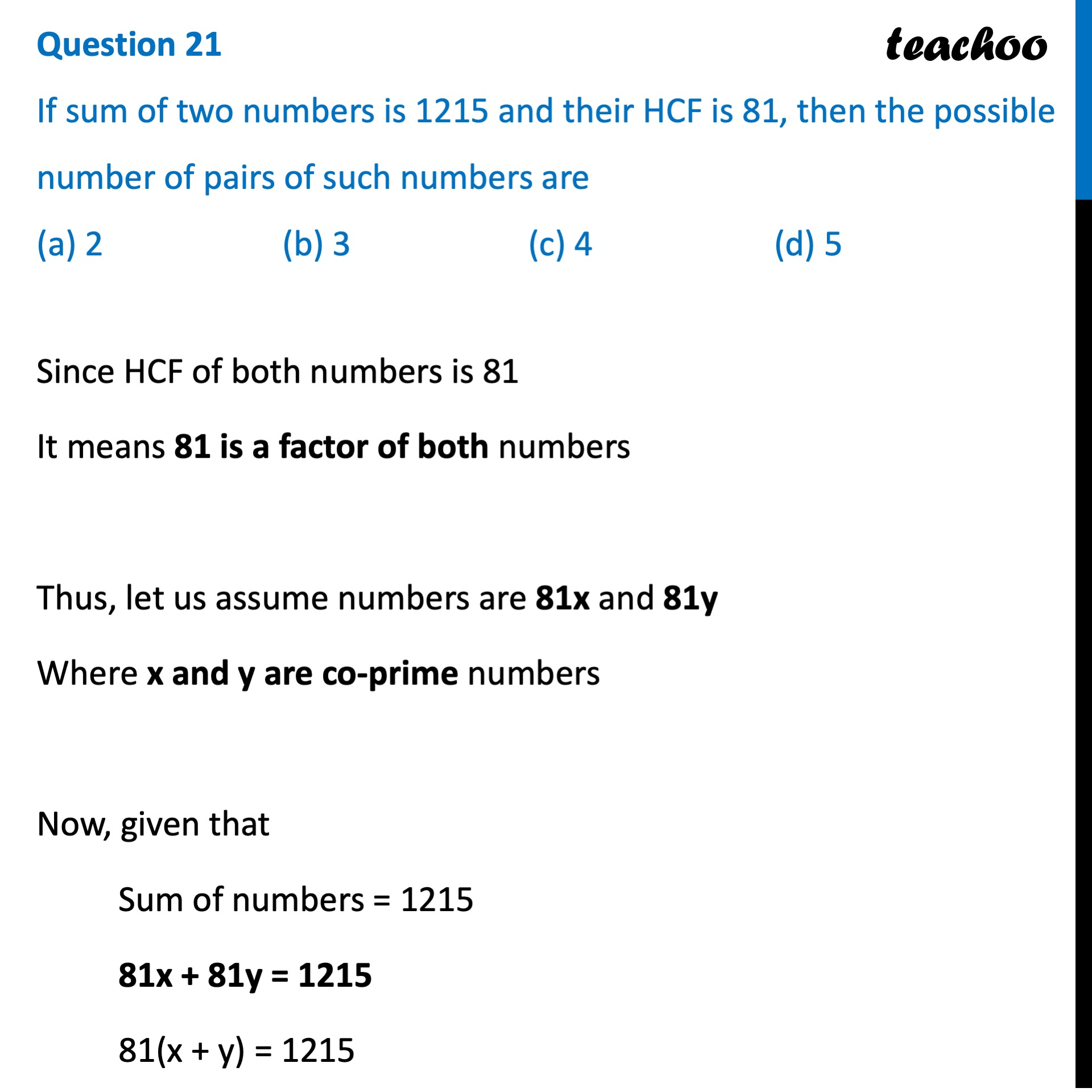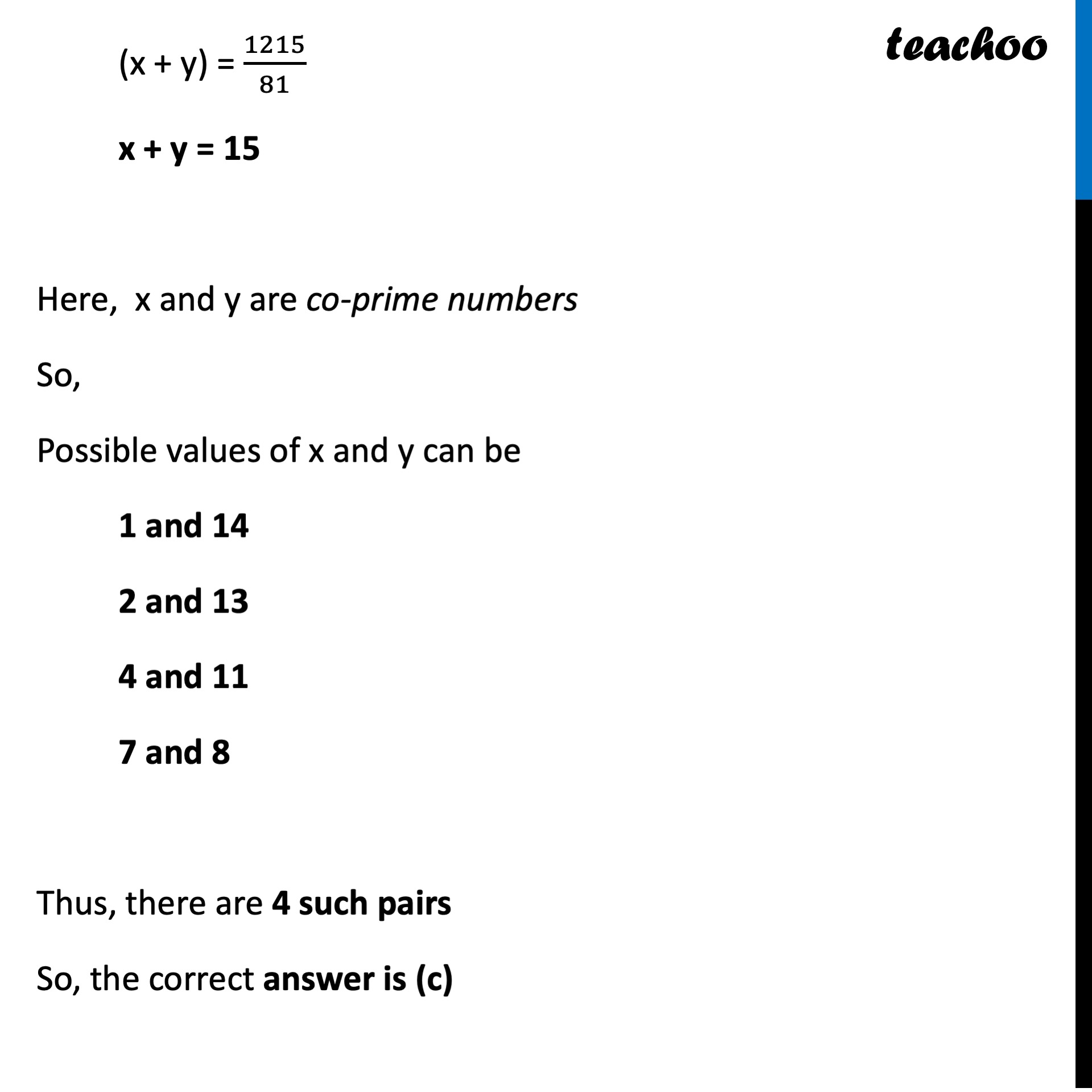CBSE Class 10 Sample Paper for 2022 Boards - Maths Standard [MCQ]

Class 10
Solutions of Sample Papers for Class 10 Boards

## (a) 2   (b) 3   (c) 4   (d) 5Learn in your speed, with individual attention - Teachoo Maths 1-on-1 Class

### Transcript

Question 21 If sum of two numbers is 1215 and their HCF is 81, then the possible number of pairs of such numbers are (a) 2 (b) 3 (c) 4 (d) 5 Since HCF of both numbers is 81 It means 81 is a factor of both numbers Thus, let us assume numbers are 81x and 81y Where x and y are co-prime numbers Now, given that Sum of numbers = 1215 81x + 81y = 1215 81(x + y) = 1215 (x + y) = 1215/81 x + y = 15 Here, x and y are co-prime numbers So, Possible values of x and y can be 1 and 14 2 and 13 4 and 11 7 and 8 Thus, there are 4 such pairs So, the correct answer is (c)